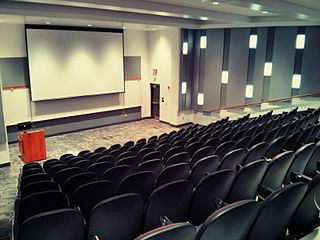# Must be a popular lecturerSuppose a lecture hall has a rectangular array of chairs with $r$ rows and $c$ columns. Suppose further that there are precisely $19$ girls seated in each row and precisely $15$ boys seated in each column.

If exactly $14$ chairs are empty then find the minimum possible value of $r \times c.$

Details and Assumptions:

• A maximum of one person per chair is allowed.
×